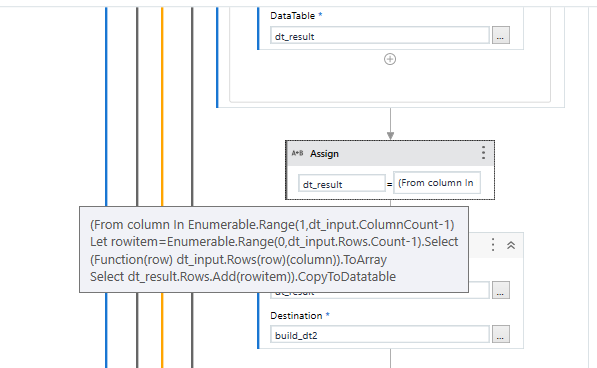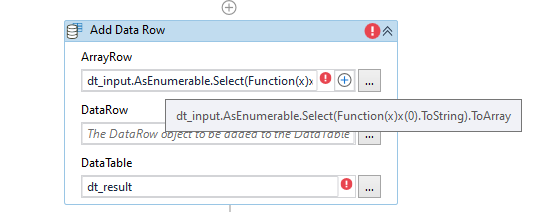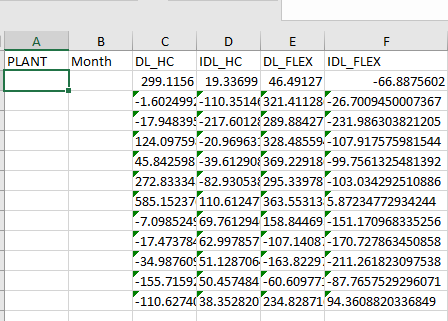# Use if condition

How to use if condition to write in excel sheets when it shows -Add Data Column: A column named ‘0’ already belongs to this Data Table.

Hi @guptasweb ,

Are you trying to catch the error and write a Value to the excel based on that error ? Is this the approach that you would really want or Could we try avoiding the error that you receive if that is possible to do.

Let us know what is the exact operation you would want to do.

then use for use to loop the table

inside the loop use instead of column name use column index (so you dont have to use the column name)

I want to extract the data from the excel sheet but it shows me this error

So in order to remove this error Can I use If condition?

The error specifically says that When Trying to Add a Data Column to the Datatable, a column with the same name already exists. So we cannot add the same column name.

Maybe an approach towards How that is happening / why is the same column name being used could be discussed and then perform an alternate approach or a different column name.

Acutually,this error comes after transposing the data in this sheet.

can you share your entire work flow as a screenshot

1 Like

hello, if you are doing a transpose operation, can you try this way by giving your own column names?
ProjeTransposeExcel.xaml (10.2 KB)You can also try this, you can rename Column A after deleting it. For this, you can write Dt.Columns(“Column0”).ColumnName=“New Name” inside the invoke code activity

could you pls tell me in my above code where I can do the enhancement?

You can change the name of the columns after the first read range operation.

We could make the Changes starting from here,

Instead of using the First Column Values as the Column Names, we will just assign Incremental integer values as the Column Names.
So, In the for Each we could use This Expression instead of Current Expression used in `In` field.

``````Enumerable.Range(0,dt_input.Rows.Count)
``````

After this use an `Add Data Row` activity and add the First Row values as the column names using the Expression below :

``````dt_input.AsEnumerable.Select(Function(x)x(0).ToString).ToArray
``````The remaining operations or steps should be the same as you have used.

Do let us know if you could make these changes and test the workflow.

Yes I can do this changes

1 LikeHow to add values in Plant column from sheetname?

where to use add data row activity in for each loop of Add column activity?

When I tested it, I am getting this output-

From G1 cell-J1 cell it wrote-0,1,2,3 instead of values.

`Add Data Row` Activity should be used Outside of `For Each` of `Add Data Column` Activity.

1 Like

I have used it but it create same file

Could you show us the Data before the Transpose ?

1 Like